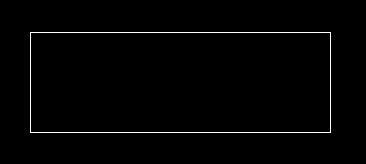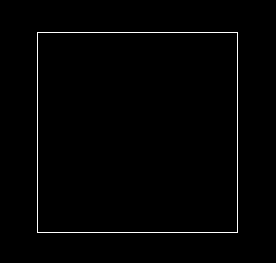Related Articles
Draw Rectangle in C graphics
• Difficulty Level : Medium
• Last Updated : 04 Oct, 2018

rectangle() is used to draw a rectangle. Coordinates of left top and right bottom corner are required to draw the rectangle. left specifies the X-coordinate of top left corner, top specifies the Y-coordinate of top left corner, right specifies the X-coordinate of right bottom corner, bottom specifies the Y-coordinate of right bottom corner.
Syntax :

```rectangle(int left, int top, int right, int bottom);
```

Examples:

```Input : left = 150, top = 250, right = 450, bottom = 350;
Output :Input : left = 150, top = 150, right = 450, bottom = 450;
Output :```

## Recommended: Please try your approach on {IDE} first, before moving on to the solution.

Below is the implementation of the rectangle function :

 `// C program to draw a rectangle``#include `` ` `// Driver code``int` `main()``{``    ``// gm is Graphics mode which is a computer display``    ``// mode that generates image using pixels.``    ``// DETECT is a macro defined in "graphics.h" header file``    ``int` `gd = DETECT, gm;`` ` `    ``// location of left, top, right, bottom``    ``int` `left = 150, top = 150;``    ``int` `right = 450, bottom = 450;`` ` `    ``// initgraph initializes the graphics system``    ``// by loading a graphics driver from disk``    ``initgraph(&gd, &gm, ``""``);`` ` `    ``// rectangle function``    ``rectangle(left, top, right, bottom);`` ` `    ``getch();`` ` `    ``// closegraph function closes the graphics``    ``// mode and deallocates all memory allocated``    ``// by graphics system .``    ``closegraph();`` ` `    ``return` `0;``}`

Output:

``````
Want to learn from the best curated videos and practice problems, check out the C Foundation Course for Basic to Advanced C.
My Personal Notes arrow_drop_up